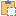#### BoundaryConditions Class

An object that represents a force applied across an area.

Namespace: Autodesk.Revit.DB.Structure
Assembly: RevitAPI (in RevitAPI.dll) Version: 21.0.0.0 (21.1.1.109)

# Syntax

C#
``public class BoundaryConditions : Element``
Visual Basic
``````Public Class BoundaryConditions _
Inherits Element``````
Visual C++
``public ref class BoundaryConditions : public Element``

# ExamplesCopy C#
``````public void GetInfo_BoundaryConditions(BoundaryConditions boundaryConditions)
{
string message = "BoundaryConditions : ";

boundaryConditions.GetBoundaryConditionsType();
switch (boundaryConditions.GetBoundaryConditionsType())
{
case BoundaryConditionsType.Point:
XYZ point = boundaryConditions.Point;
message += "\nThis BoundaryConditions is a Point Boundary Conditions.";
message += "\nLocation point: (" + point.X + ", "
+ point.Y + ", " + point.Z + ")";
break;
case BoundaryConditionsType.Line:
message += "\nThis BoundaryConditions is a Line Boundary Conditions.";
Curve curve = boundaryConditions.GetCurve();
// Get curve start point
message += "\nLocation Line: start point: (" + curve.GetEndPoint(0).X + ", "
+ curve.GetEndPoint(0).Y + ", " + curve.GetEndPoint(0).Z + ")";
// Get curve end point
message += ";  end point:(" + curve.GetEndPoint(1).X + ", "
+ curve.GetEndPoint(1).Y + ", " + curve.GetEndPoint(1).Z + ")";
break;
case BoundaryConditionsType.Area:
message += "\nThis BoundaryConditions is an Area Boundary Conditions.";
IList<CurveLoop> loops = boundaryConditions.GetLoops();
foreach (CurveLoop curveLoop in loops)
{
foreach (Curve areaCurve in curveLoop)
{
// Get curve start point
message += "\nCurve start point:(" + areaCurve.GetEndPoint(0).X + ", "
+ areaCurve.GetEndPoint(0).Y + ", " + areaCurve.GetEndPoint(0).Z + ")";
// Get curve end point
message += "; Curve end point:(" + areaCurve.GetEndPoint(1).X + ", "
+ areaCurve.GetEndPoint(1).Y + ", " + areaCurve.GetEndPoint(1).Z + ")";
}
}
break;
default:
break;
}

}``````Copy VB.NET
``````Public Sub GetInfo_BoundaryConditions(boundaryConditions As BoundaryConditions)
Dim message As String = "BoundaryConditions : "

boundaryConditions.GetBoundaryConditionsType()
Select Case boundaryConditions.GetBoundaryConditionsType()
Case BoundaryConditionsType.Point
Dim point As XYZ = boundaryConditions.Point
message += vbLf & "This BoundaryConditions is a Point Boundary Conditions."
message += vbLf & "Location point: (" + point.X + ", " + point.Y + ", " + point.Z + ")"
Exit Select
Case BoundaryConditionsType.Line
message += vbLf & "This BoundaryConditions is a Line Boundary Conditions."
Dim curve As Curve = boundaryConditions.GetCurve()
' Get curve start point
message += vbLf & "Location Line: start point: (" + curve.GetEndPoint(0).X + ", " + curve.GetEndPoint(0).Y + ", " + curve.GetEndPoint(0).Z + ")"
' Get curve end point
message += ";  end point:(" + curve.GetEndPoint(1).X + ", " + curve.GetEndPoint(1).Y + ", " + curve.GetEndPoint(1).Z + ")"
Exit Select
Case BoundaryConditionsType.Area
message += vbLf & "This BoundaryConditions is an Area Boundary Conditions."
Dim loops As IList(Of CurveLoop) = boundaryConditions.GetLoops()
For Each curveLoop As CurveLoop In loops
For Each areaCurve As Curve In curveLoop
' Get curve start point
message += vbLf & "Curve start point:(" + areaCurve.GetEndPoint(0).X + ", " + areaCurve.GetEndPoint(0).Y + ", " + areaCurve.GetEndPoint(0).Z + ")"
' Get curve end point
message += "; Curve end point:(" + areaCurve.GetEndPoint(1).X + ", " + areaCurve.GetEndPoint(1).Y + ", " + areaCurve.GetEndPoint(1).Z + ")"
Next
Next
Exit Select
Case Else
Exit Select
End Select